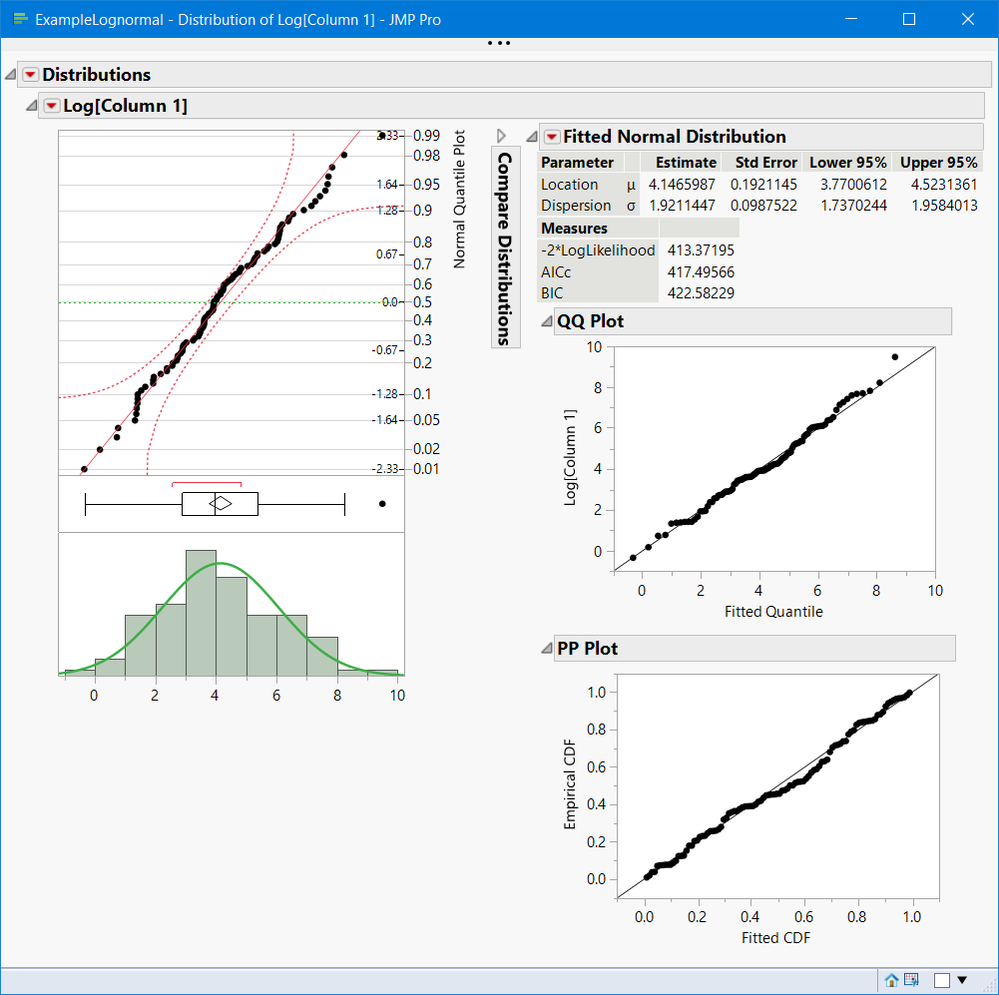Our World Statistics Day conversations have been a great reminder of how much statistics can inform our lives. Do you have an example of how statistics has made a difference in your life? Share your story with the Community!
Choose Language Hide Translation Bar
Highlighted

## Probability plot for log-normal distribution fitting

Hi,

Is there an option to view the probability plot when making a log-normal fit with a distribution analysis?

Thanks

2 ACCEPTED SOLUTIONS

Accepted Solutions
Highlighted

## Re: Probability plot for log-normal distribution fitting

Hi,

From the red hotspot of beside the name of your column in the Distribution platform choose "Continuous Fit -->Fit lognormal."  Then, from red hotspot beside "Fitted Lognormal Distribution," choose "Diagnostic Plots."  You then have the option of fitting the P-P and/or Q-Q plots.  Is that what you're looking for?

Highlighted

## Re: Probability plot for log-normal distribution fitting

@HadleyMyers provided you with a solution. However, if your lognormal distribution has a large mean and/or variance, the scaling on the QQ plot usually does not have the familiar custom probability plot that scales the Y axes like custom probability paper (yes I am showing my age, things we used pre graphical calculator/computer days).

I suggest you right click on the column of data you're fitting, select Transform and use Log, then Continuous Fit >  Fit Normal.

Below is a screenshot of  Analyze > Distribution > Continuous Fit > Fit Normal of 100 random lognormal(4,2) values transformed with Log. The table with script is attached.

Another suggestsion is to use the QQ plot that Hadley suggested and click on the Y axis of he QQ plot and change Linear to Log.4 REPLIES 4
Highlighted

## Re: Probability plot for log-normal distribution fitting

Hi,

From the red hotspot of beside the name of your column in the Distribution platform choose "Continuous Fit -->Fit lognormal."  Then, from red hotspot beside "Fitted Lognormal Distribution," choose "Diagnostic Plots."  You then have the option of fitting the P-P and/or Q-Q plots.  Is that what you're looking for?

Highlighted

## Re: Probability plot for log-normal distribution fitting

@HadleyMyers provided you with a solution. However, if your lognormal distribution has a large mean and/or variance, the scaling on the QQ plot usually does not have the familiar custom probability plot that scales the Y axes like custom probability paper (yes I am showing my age, things we used pre graphical calculator/computer days).

I suggest you right click on the column of data you're fitting, select Transform and use Log, then Continuous Fit >  Fit Normal.

Below is a screenshot of  Analyze > Distribution > Continuous Fit > Fit Normal of 100 random lognormal(4,2) values transformed with Log. The table with script is attached.

Another suggestsion is to use the QQ plot that Hadley suggested and click on the Y axis of he QQ plot and change Linear to Log.Highlighted

## Re: Probability plot for log-normal distribution fitting

Hi gzmorgan0,
I agree, making the log transformation is a great way to get the intervals on the plot too. I did this before but was also wondering about getting a plot for the log fit. The PP/QQ do a good job.
Thanks for the help.
Highlighted